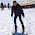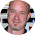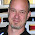This weekend, I create a Valentine for my wife, using Tableau, which visualized each of the 6,542 days we’ve been together (yeah, I know that’s totally nerdy, but I thought it was a bit more creative than a card or chocolates). The visualization took the form of a heart made up of individual dots, each dot representing one day of our relationship. Here’s the visualization:

I don’t know that there’s much utility to such a visualization, other than creating Valentines, but it was fun to create and it stretched my skills a bit, so I decided to write up a brief How-To.

A Couple of Disclaimers

1.       Though it is possible to perform the calculations in Tableau and there are some compelling reasons to do so (see the second disclaimer), I decided to calculate my points completely in Excel. There were two drivers for this. First, I was looking to plot individual dots representing each day of our relationship, so there is some additional data tied to each point. Second, as a data architect, I often like to take a tool agnostic approach to data preparation. So, while I’m using Tableau, you could easily use any other tool that allows you to plot coordinates.

2.      After writing this, I saw a similar post from Jeffrey A. Shaffer, Drawing Shapes in Tableau, which describes the creation of a heart shape (and other shapes) in a much more succinct manner than the rambling, stream-of-consciousness post you’re about to read. Our approaches use the same math, but his is way less complex and would probably be a better option for most use cases.

Creating the Heart
A heart shape is nothing more than two mirrored curves meeting in the middle. So, like other curves, I figured there must be a mathematical equation to describe them. And, as detailed on Wolfram Math World, there is. The curves are described parametrically as follows:

x = 16 sin3 t
y = 13 cos t - 5 cos (2 t) - 2 cos (3 t) - cos (4 t)

I do not remember ever learning about parametric equations, despite the three years I spent in Calculus courses. So, if you’re like me, you may need a brief refresher. I found a Paul’s Online Math Notes. Here’s a brief excerpt:

There are also a great many curves out there that we can’t even write down as a single equation in terms of only x and y. So, to deal with some of these problems we introduce parametric equations. Instead of defining y in terms of x (  ) or x in terms of y (  ) we define both x and y in terms of a third variable called a parameter as follows,

y = f ( t )                    y = g ( t )

This third variable is usually denoted by t…Sometimes we will restrict the values of t that we’ll use and at other times we won’t. This will often be dependent on the problem and just what we are attempting to do.

Each value of t defines a point  that we can plot. The collection of points that we get by letting t be all possible values is the graph of the parametric equations and is called the parametric curve.

Got it? It took me a bit, but it finally sunk in. But how do we figure out what values to use for t? Well, I started by just picking some numbers. I decided to start by plotting 50 points, so I chose t values ranging from -25 to 24. I then used Excel to calculate the values of x and y based on the equations above. From there, I plotted the data in Tableau.

Not bad for a first attempt! It definitely has the shape of a heart. But, as you can clearly see, picking random values for t was not going to give me evenly spaced dots. One option was to keep guessing values and, through trial and error, we might eventually land on values that get close to evenly spaced. But that seemed like way too much work and it was not an automated solution, so I quickly rejected the idea. Instead, I started doing some additional reading on the heart curve to see what more I could learn. I was thrilled when I came upon a MATLAB community discussion on the MathWorks website, which provided a method for calculating values of t which would create evenly spaced dots.

t = linspace(-π, π, 350)

If you’re familiar with MATLAB or languages such as Python or R, then you’ve probably worked with linspace. For the uninitiated, linspace “returns a row vector of…evenly spaced points between” two numbers. In our example, these two numbers are -π and π. The third parameter of the function defines the number of points you wish to generate (For more details, see the MATLAB documentation).

I’ve dabbled  with Python and R, but my skills are limited. And I really didn’t want to switch over to one of these just to calculate a few values. Luckily for me, I found a post on pfadintegral.com, which included an Excel VBA version of the linspace function. So, I saved my data as a macro-enabled spreadsheet and copied over the linspace code. I then used it to calculate values for t. A couple of notes on this step:

• Though the formula above suggest 350 as the number of points, this is entirely up to you. I chose to go with 200 points.
• The linspace function returns an array of values, so it must be entered as an array formula in Excel. If you’re not familiar with this, you can read more about it here.

Now armed with 200 points which should be evenly spaced, I used Tableau to visualize them.
We’re getting there…The points do overlap a bit in tighter spaces, but I was okay with that and thought it added a bit of a nice effect. But, at this point, I only had a single outline of a heart, made up of 200 dots. Since I needed a dot for 6,542 days, I decided to create additional inner hearts of 200 dots each. To do this, I would need 32 “rings” of 200 dots each and a 33rd ring with the leftover 142 dots. In my spreadsheet, I created the 6,542 rows of data, copied all of my formulas, then separated them into the 33 different rings (via a “Ring Number” column). However, without changing the formulas, the calculated t, x, and y values were all the same. So, for each subsequent ring, I reduced the x and y values by 1/33. So, the second ring is 1/33 smaller than the first ring and the third ring was 2/33 smaller than the first, and so on, until the final ring was 32/33 smaller than the first ring. I then visualized in Tableau.

One obvious problem is that each ring has the same number of dots, but the inner rings have increasingly less space to put them. I considered reducing the number of dots in the inner rings, but after looking at this for a while, I have to admit that I quite like the effect of it and really didn’t feel it was necessary to take that step. I did, however, adjust the dot size slightly according to the ring number, so that the dots grow smaller as you move inward.

So, there you have it. Creating a heart is relatively easy, if you can get over the somewhat complex math. If you’d like to create one of your own, I certainly hope this post helps. I’ve uploaded a copy of my Excel template, which you can find here. You can also download a copy of the Tableau dashboard on Tableau Public.

Ken Flerlage, February 13, 2017

1.It's amazing work!

1.Thanks Andy!

2.Amazing. I should create one for my own.
Thank you, Ken!

1.Thank you. If you do create one, be sure to share it with me. I'd love to see it!

3.very nice visualization. I am trying other shapes in tableau now. Thanks for the inspiring work

1.Fantastic. I'd love to see what you create!

4.Nice visualization. Trying other shapes in tableau. Inspiring work.

5.Your mode of telling the whole thing in this post is truly nice, every one can easily be aware of it, Thanks a lot. outlook 365 login